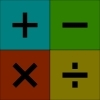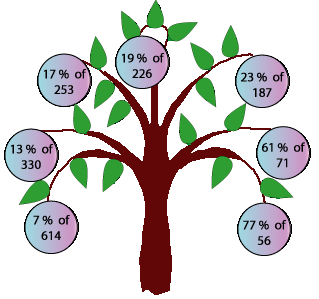#### You may also like### Worms

Place this "worm" on the 100 square and find the total of the four squares it covers. Keeping its head in the same place, what other totals can you make?### Which Scripts?

There are six numbers written in five different scripts. Can you sort out which is which?### Highest and Lowest

Put operations signs between the numbers 3 4 5 6 to make the highest possible number and lowest possible number.

# The Magic Number and the Hepta-tree

## The Magic Number and the Hepta-Tree

The Mathemagician needs to break a dreadful spell! Help him to find the magic number that will do the trick!

Here is the hepta-tree hung with silver balls.Do you think the balls will have similar values?

Try estimating the amount on each ball.

In order to break the spell, firstly find the exact difference between the largest ball and the smallest ball.

Multiply your result by $244$.

Round this to a whole number and that gives us the MAGIC NUMBER!

Give this number to the Mathemagician and the terrible spell will be broken for ever!

### Why do this problem?

This problem gives work on percentages in an interesting and challenging way. It also gives you an opportunity to discuss different ways of estimating and therefore shows learners how expressions which look very different can give very close answers.

### Possible approach

You could start by showing the group the picture of the hepta-tree and invite them to give a quick response as to which ball they think has the highest value and which one the smallest. Ask them to estimate the value of each ball in pairs and encourage several pairs to share their method with everyone. This will bring out the fact that actually the balls have very similar values.

After this they could work in pairs on the problem itself, possibly using this sheet (which lists the expressions on the balls). Learners are likely to need pencil and paper or a mini-whiteboard and pen, and possibly a calculator.

At the end of the lesson encourage the group to discuss the problem. Finding out how each pair approached it could be very useful as learners will gain from seeing what others have done. By sharing calculation strategies, children's understanding of the relationship between percentages, fractions and decimals will be deepened and you will be able to assess how comfortable they are with this area of mathematics.

### Key questions

What do you notice when you estimate the value of each ball?
How could you work out the exact value of this ball?
What do you notice about the numbers you have found?
How do you know which is the highest/lowest number?

### Possible extension

Learners could create more percentages like the ones on the hepta-tree which look different but have very close answers. These could range like the ones in the problem or approximate to another number. It would be interesting to talk to children about how they were coming up with the percentages.

### Possible support

Using this sheet will help children organise their calculations and it may be appropriate for some to use a calculator.